# Solid geometry, stereometry

Solid geometry is the name for the geometry of three-dimensional Euclidean space.

Stereometry deals with the measurements of volumes of various solid figures (three-dimensional figures) including pyramids, prisms and other polyhedrons; cylinders; cones; truncated cones; and balls bounded by spheres.

1. Surface of the cylinderCalculate the surface area of the cylinder when its volume is 45 l and the perimeter of base is three times of the height.
2. Volume and surfaceCalculate the volume and surface area of the cylinder when the cylinder height and base diameter is in a ratio of 3:4 and the area of the cylinder jacket is 24 dm2.
3. Tin with oilTin with oil has the shape of a rotating cylinder whose height is equal to the diameter of its base. Canned surface is 1884 cm2. Calculate how many liters of oil is in the tin.
4. The cylindrical containerThe container has a cylindrical shape the base diameter 0.8 meters has a content area of the base is equal to the content area of the shell. How many full liters of water can be poured maximally into the container?
5. Fire tankHow deep is the fire tank with the dimensions of the bottom 7m and 12m, when filled with 420 m3 of water?
6. Juice box 2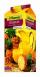Box with juice has the shape of a cuboid. Internal dimensions are 15 cm, 20 cm and 32 cm. If the box stay at the smallest base juice level reaches 4 cm below the upper base. How much internal volume of the box fills juice? How many cm below the top of th
7. Max - cone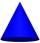From the iron bar (shape = prism) with dimensions 6.2 cm, 10 cm, 6.2 cm must be produced the greatest cone. a) Calculate cone volume. b) Calculate the waste.
8. Water levelHow high reaches the water in the cylindrical barell with a diameter of 12 cm if there is a liter of water? Express in cm with an accuracy of 1 decimal place.
9. Barrel 3Barrel with water has a weight 118 kg. When we get off 75% of water it has a weight 35 kg. How many kg has empty barrel?
10. Truck of milk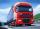How many hectoliters of ,,the box" milk fit in the truck, ake cargo size area is 2.8 m x 3 m x 17 m? A liter of milk in a box measuring 12 cm x 7 cm x 20 cm.
11. Garden pool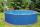Mr. Novak fill garden pool (cylinder; diameter of 200 cm) with 31.4 hl of water. What is the depth of the pool when the water level is 10 cm below the upper edge of the pool?
12. Water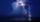On the lawn which has area 914 m2 rained 3 mm of water. How many liters of water rained?
13. CuboidHow many times will increase the volume of a cuboid, if one dimension is twice larger, second dimension three times larger and third dimension four times lower?
14. GreenhouseGarden plastic greenhouse is shaped half cylinder with a diameter of 6 m and base length 20 m. At least how many m2 of plastic is need to its cover?
15. Bottles of juice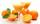How many 2-liter bottles of juice need to buy if you want to transfer juice to 50 pitchers rotary cone shape with a diameter of 24 cm and base side length of 1.5 dm.
16. Wood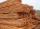Wood contains 12% water. 1 m3 of wood weighs 650 kg. How many liters of water it contains?
17. Weight of airWhat is the weight of air in the living room measuring width 10 m length 3 m and height 3.3 m? Air density is ρ = 1.2 kg/m3.
18. Cylinder diameter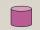The surface of the cylinder is 149 cm2. The cylinder height is 6 cm. What is the diameter of this cylinder?
19. Cuboid - edgesThe sum of all edges cuboid are 8 meters. However, the width is twice shorter than the length and height is seven times longer than the width. Determine the dimensions of the cuboid.
20. Cuprum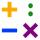From 1600 mm long sheet of copper plate 2 mm thickness we have separated over the whole length the belt weighing 6000 g. Calculate belt width if 1 dm3 copper weighs 8.9 kg.

Do you have an interesting mathematical word problem that you can't solve it? Submit math problem, and we can try to solve it.

We will send a solution to your e-mail address. Solved examples are also published here. Please enter the e-mail correctly and check whether you don't have a full mailbox.

Please do not submit problems from current active competitions such as Mathematical Olympiad, correspondence seminars etc...